# Given two lines in​ space, either they are​ parallel, they​ intersect, or they are skew​ (lie in parallel​ planes). Dete...

Given two lines in​ space, either they are​ parallel, they​ intersect, or they are skew​ (lie in parallel​ planes). Determine whether the lines​ below, taken two at a​ time, are​ parallel, intersect, or are skew. If they​ intersect, find the point of intersection.​ Otherwise, find the distance between the two lines.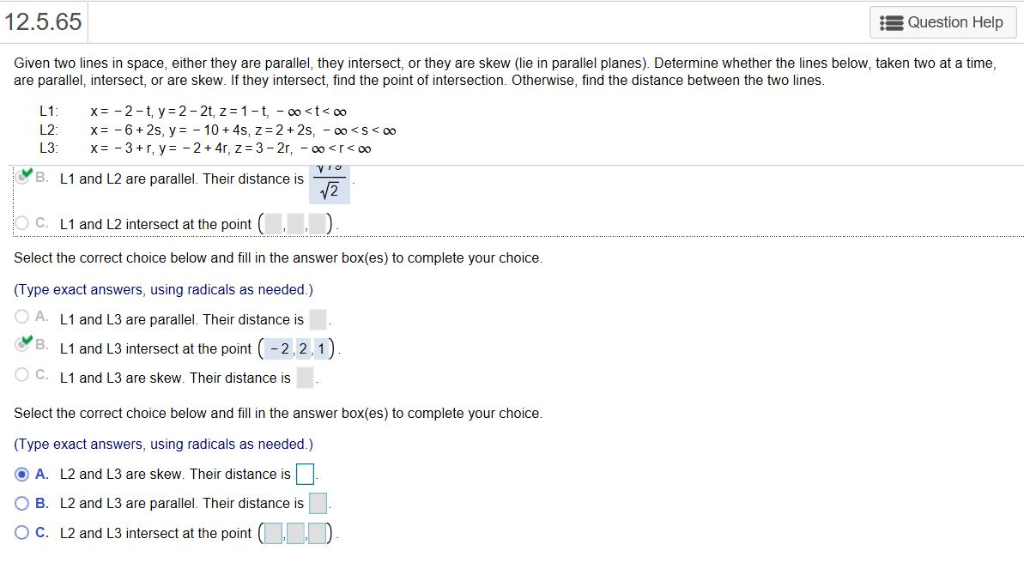12.5.65 Question Help Given two lines in space, either they are parallel, they intersect, or they are skew (lie in parallel planes). Determine whether the lines below, taken two at a time are parallel, intersect, or are skew. If they intersect, find the point of intersection. Otherwise, find the distance between the two lines B. L1 and L2 are parallel. Their distance is C. L1 and L2 intersect at the point Select the correct choice below and fill in the answer box(es) to complete your choice (Type exact answers, using radicals as needed.) 0 A. L1 and L3 are parallel Their distance is B. L1 and L3 intersect at the point (-2.2.1) O C. L1 and L3 are skew. Their distance is Select the correct choice below and fill in the answer box(es) to complete your choice (Type exact answers, using radicals as needed.) @a. L2 and L3 are skew. Ther distance is □ O B. L2 and L3 are parallel. Their distance is ° C. L2 and L3 intersect at the point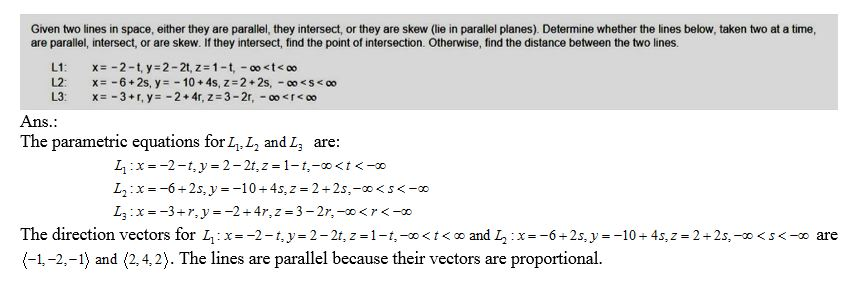##### Add Answer of: Given two lines in​ space, either they are​ parallel, they​ intersect, or they are skew​ (lie in parallel​ planes). Dete...
Similar Homework Help Questions
• ### Given two lines in space, either they are parallel, they intersect, or they are skew (lie in para...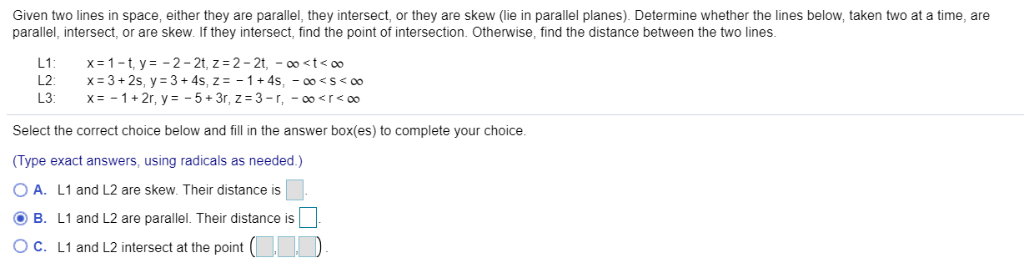The same question but has 3 parts Given two lines in space, either they are parallel, they intersect, or they are skew (lie in parallel planes). Determine whether the lines below, taken two at a time, are parallel, intersect, or are skew. If they intersect, find the point of intersection. Otherwise, find the distance between the two lines. Select the correct choice below and fill in the answer box(es) to complete your choice. Type exact answers, using radicals as needed.)...

• ### Determine if two lines are parallel;, skew, intersecting...

I'm trying to find of two lines are parallel,skew, or intersecting. If they intersect, find the point of intersection; if they are skewed, find the distance betweenthem.x= 1-2t x= -7+8tl1: y= 3+4t l2:y= 9tz= -5-6t z= 10

• ### Lines and Planes in Space

Consider the lines,L1: x=5+2s, y= -2+s, z=1-3sL2: x=2t, y=2-t, z= -1+tAre they parallel? orthogonal? skew?justify answers in as much detail as possible.If the lines intersect, find their point of intersection.Thanks.

• ### Skew lines 3

Consider the following two lines in space.L1 : x=4 + 4t, y = 5 + 5t, z = 1- 4tL2 : x=4 + s, y= -6 + 8s, z = 7 - 3sfind the distance between the parallel planes. This is the distance between the two skew lines

• ### Determine if each pair of lines are parallel, skew or intersecting. If the lines intersect, find the point of intersection. Otherwise, find the distance between the lines. Then find a point on each li...

Determine if each pair of lines are parallel, skew or intersecting. If the lines intersect, find the point of intersection. Otherwise, find the distance between the lines. Then find a point on each line such that the distance between the points is the distance between the lines. Draw a picture, and use vectors instead of distance formulas to find the distance. Line #1 = < -2,2,8> + t< 1,2,2> Line#2 = < 0,1,5 > + t< -2,-4, -4>

• ### A student claims that if any two planes that do not intersect are parallel, then any two lines that do not intersect should also be parallel

A student claims that if any two planes that do not intersectare parallel, then any two lines that do not intersect shouldalso be parallel. How do you respond?

• ### Question (2): (5 Marks) x-1-3-y x-1-6-y:+2 are (A) Determine intersecting or skew. If they intersect, find the point of intersection Given SI: x2-2y2 = 4z2-252 &s2: (0 Show that the tangent p...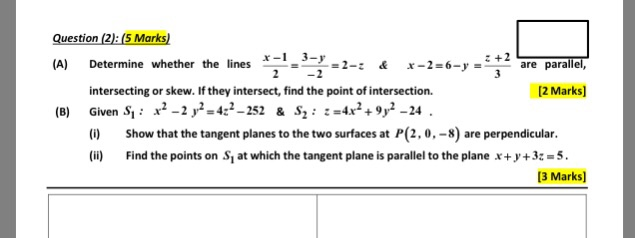Question (2): (5 Marks) x-1-3-y x-1-6-y:+2 are (A) Determine intersecting or skew. If they intersect, find the point of intersection Given SI: x2-2y2 = 4z2-252 &s2: (0 Show that the tangent planes to the two surfaces at P(2,0,-8) are perpendicular. whether the lines parallel, 2-z & 12 Marks] 4x2 +9y2-24. (B) Find the points on Si at which the tangent plane is parallel to the plane x+y+32-5 3 Marks] Question (2): (5 Marks) x-1-3-y x-1-6-y:+2 are (A) Determine intersecting or...

• ### Please tell me which options I need to select and what I have to type in. Thank you! 3-3x For the given rational function f(x)- x- find the following (A) Find the intercepts for the graph. (B) Determi...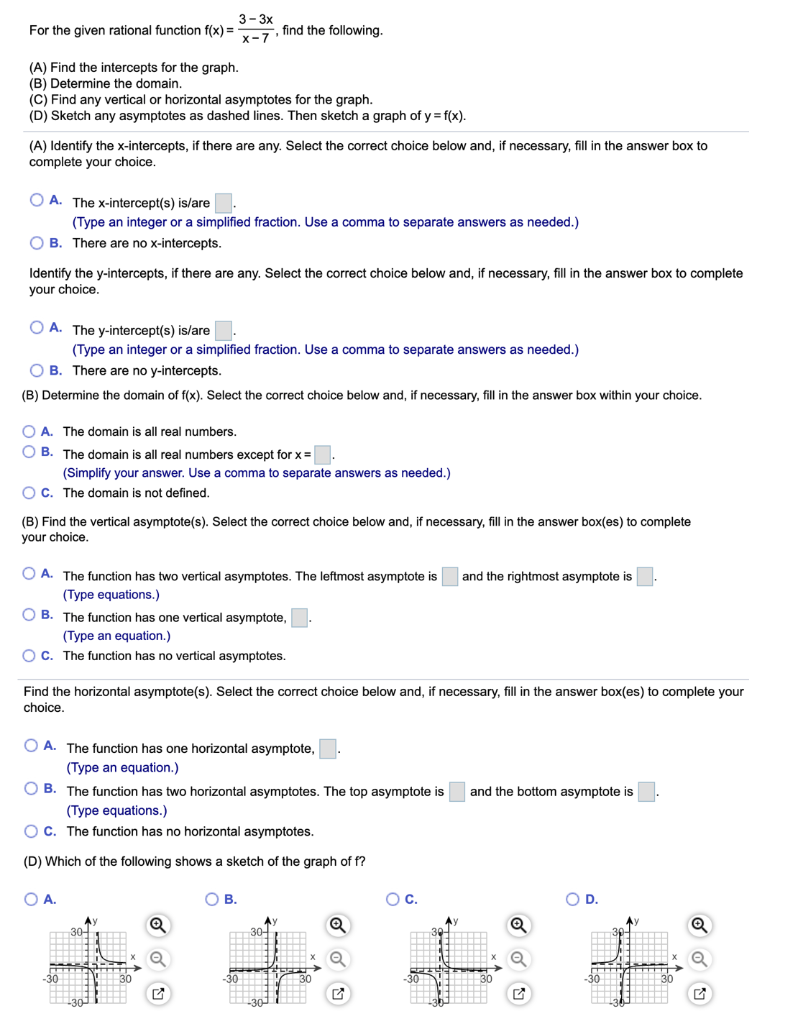Please tell me which options I need to select and what I have to type in. Thank you! 3-3x For the given rational function f(x)- x- find the following (A) Find the intercepts for the graph. (B) Determine the domain. (C) Find any vertical or horizontal asymptotes for the graph (D) Sketch any asymptotes as dashed lines. Then sketch a graph of y f(x) (A) Identify the x-intercepts, if there are any. Select the correct choice below and, if necessary,...

• ### Two sociologists have grant money to study school busing in a particular city. They wish to...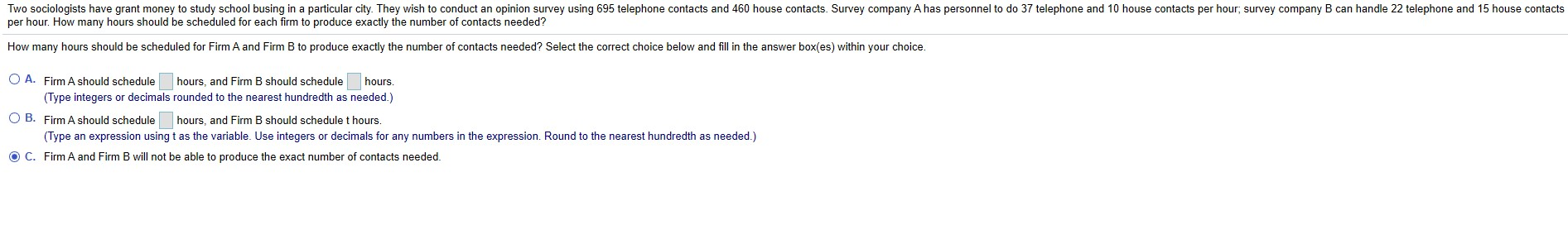Two sociologists have grant money to study school busing in a particular city. They wish to conduct an opinion survey using 695 telephone contacts and 460 house contacts. Survey company A has personnel to do 37 telephone and 10 house contacts per hour, survey company B can handle 22 telephone and 15 house contacts per hour. How many hours should be scheduled for each firm to produce exactly the number of contacts needed? How many hours should be scheduled for...

• ### A. Determine whether the Mean Value Theorem applies to the function fx)xon the interval [3,7 b. I...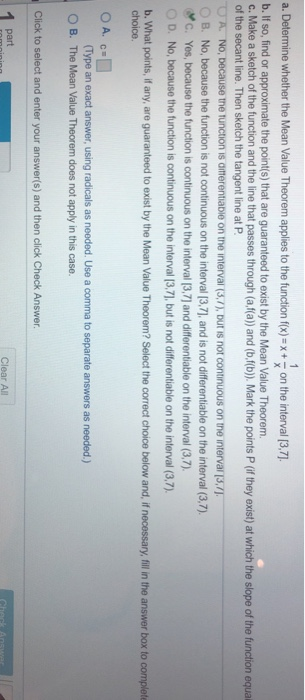a. Determine whether the Mean Value Theorem applies to the function fx)xon the interval [3,7 b. If so, find or approximate the point(s) that are guaranteed to exist by the Mean Value Theorem. c. Make a sketch of the function and the line that passes through (a,f(a) and (b.f(b). Mark the points P (if they exist) at which the slope of the function equali of the secant line. Then sketch the tangent line at P A. No, because the tunction...

Need Online Homework Help?